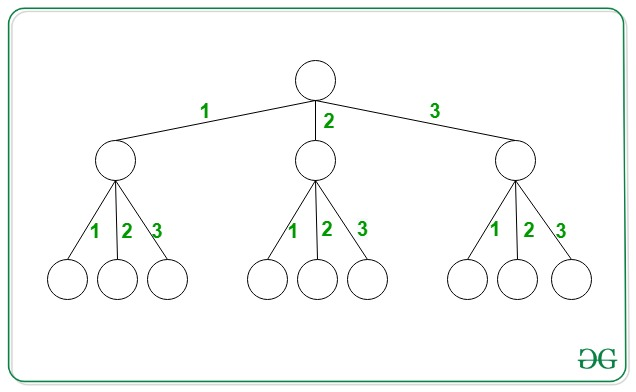# Count unique paths with given sum in an N-ary Tree

• Difficulty Level : Medium
• Last Updated : 01 Oct, 2021

Given an integer X and integer N, the task is to find the number of unique paths starting from the root in N-ary tree such that the sum of all these paths is equal to X. The N-ary tree satisfies the following conditions:

• All the nodes have N children and the weight of each edge is distinct and lies in the range [1, N].
• The tree is extended up to infinity.

Examples:

Input: N = 3, X = 2Output: 2
Explanation: the two paths having path sum equal to 2 are {1, 1} and {2}.

Input: N = 3, X = 6
Output: 24

Naive Approach: The simplest approach is to recursively find all possible ways to obtain path sum equal to X and print the count obtained.

Time Complexity: O(N * X)
Auxiliary Space: O(1)

Efficient Approach: To optimize the above approach, the idea is to use Dynamic Programming. Follow the steps below to solve the problem:

• Initialize a dp[] array which for every ith index, stores the count of paths adding up to i.
• For every vertex, iterate form strong>1 to min(X, N), i.e. all possible values of its children and find the number of paths possible with given sum from each node considered.
• Add all the paths made using the edges 1 to N and check if count is already computed or not. If already computed, return the value. Otherwise, recursively count the number of paths with sum equal to current value by considering all possible ways to extend the tree from the current vertex.
• Update the dp[] array and return the count obtained.

Below is the implementation of the above approach:

## C++

 `// C++ program for the above approach``#include ``#define ll long long``using` `namespace` `std;``const` `int` `mod = (``int``)1e9 + 7;` `// Function for counting total``// no of paths possible with``// the sum is equal to X``ll findTotalPath(``int` `X, ``int` `n,``                 ``vector<``int``>& dp)``{` `    ``// If the path of the sum``    ``// from the root to current``    ``// node is stored in sum``    ``if` `(X == 0) {``        ``return` `1;``    ``}``    ``ll ans = 0;` `    ``// If already computed``    ``if` `(dp[X] != -1) {``        ``return` `dp[X];``    ``}` `    ``// Count different no of paths``    ``// using all possible ways``    ``for` `(``int` `i = 1; i <= min(X, n); ++i) {` `        ``ans += findTotalPath(``                   ``X - i, n, dp)``               ``% mod;``        ``ans %= mod;``    ``}` `    ``// Return total no of paths``    ``return` `dp[X] = ans;``}` `// Driver Code``int` `main()``{` `    ``int` `n = 3, X = 2;` `    ``// Stores the number of ways``    ``// to obtains sums 0 to X``    ``vector<``int``> dp(X + 1, -1);` `    ``// Function call``    ``cout << findTotalPath(X, n, dp);``}`

## Java

 `// Java program for the above approach``import` `java.io.*;``import` `java.util.*;` `class` `GFG{` `static` `int` `mod = (``int``)1e9 + ``7``;` `// Function for counting total``// no of paths possible with``// the sum is equal to X``static` `int` `findTotalPath(``int` `X, ``int` `n,``                         ``ArrayList dp)``{``    ` `    ``// If the path of the sum``    ``// from the root to current``    ``// node is stored in sum``    ``if` `(X == ``0``)``    ``{``        ``return` `1``;``    ``}``    ``int` `ans = ``0``;` `    ``// If already computed``    ``if` `(dp.get(X) != -``1``)``    ``{``        ``return` `dp.get(X);``    ``}` `    ``// Count different no of paths``    ``// using all possible ways``    ``for``(``int` `i = ``1``; i <= Math.min(X, n); ++i)``    ``{``        ``ans += findTotalPath(X - i, n, dp) % mod;``        ``ans %= mod;``    ``}` `    ``// Return total no of paths``    ``dp.set(X, ans);``    ``return` `ans;``}` `// Driver Code``public` `static` `void` `main(String[] args)``{``    ``int` `n = ``3``, X = ``2``;``    ` `    ``// Stores the number of ways``    ``// to obtains sums 0 to X``    ``ArrayList dp = ``new` `ArrayList(``        ``Collections.nCopies(X + ``1``, -``1``));` `    ``// Function call``    ``System.out.print(findTotalPath(X, n, dp));``}``}` `// This code is contributed by akhilsaini`

## Python3

 `# Python3 program for the above approach``mod ``=` `int``(``1e9` `+` `7``)` `# Function for counting total``# no of paths possible with``# the sum is equal to X``def` `findTotalPath(X, n, dp):``    ` `  ``# If the path of the sum``  ``# from the root to current``  ``# node is stored in sum``  ``if` `(X ``=``=` `0``):``    ``return` `1``    ` `  ``ans ``=` `0` `  ``# If already computed``  ``if` `(dp[X] !``=` `-``1``):``    ``return` `dp[X]``    ` `  ``# Count different no of paths``  ``# using all possible ways``  ``for` `i ``in` `range``(``1``, ``min``(X, n) ``+` `1``):``    ``ans ``=` `ans ``+` `findTotalPath(X ``-` `i, n, dp) ``%` `mod;``    ``ans ``%``=` `mod;` `  ``# Return total no of paths``  ``dp[X] ``=` `ans``  ``return` `ans` `# Driver Code``if` `__name__ ``=``=` `'__main__'``:``    ` `  ``n ``=` `3``  ``X ``=` `2` `  ``# Stores the number of ways``  ``# to obtains sums 0 to X``  ``dp ``=` `[``-``1``] ``*` `(X ``+` `1``)` `  ``# Function call``  ``print``(findTotalPath(X, n, dp))``  ` `# This code is contributed by akhilsaini`

## C#

 `// C# program for the above approach``using` `System;``using` `System.Collections;``using` `System.Collections.Generic;` `class` `GFG{` `static` `int` `mod = (``int``)1e9 + 7;` `// Function for counting total``// no of paths possible with``// the sum is equal to X``static` `int` `findTotalPath(``int` `X, ``int` `n, ``int``[] dp)``{``    ` `    ``// If the path of the sum``    ``// from the root to current``    ``// node is stored in sum``    ``if` `(X == 0)``    ``{``        ``return` `1;``    ``}``    ``int` `ans = 0;` `    ``// If already computed``    ``if` `(dp[X] != -1)``    ``{``        ``return` `dp[X];``    ``}` `    ``// Count different no of paths``    ``// using all possible ways``    ``for``(``int` `i = 1; i <= Math.Min(X, n); ++i)``    ``{``        ``ans += findTotalPath(X - i, n, dp) % mod;``        ``ans %= mod;``    ``}` `    ``// Return total no of paths``    ``dp[X] = ans;``    ``return` `ans;``}` `// Driver Code``public` `static` `void` `Main()``{``    ``int` `n = 3, X = 2;``    ` `    ``// Stores the number of ways``    ``// to obtains sums 0 to X``    ``int``[] dp = ``new` `int``[X + 1];``    ``Array.Fill(dp, -1);` `    ``// Function call``    ``Console.WriteLine(findTotalPath(X, n, dp));``}``}` `// This code is contributed by akhilsaini`

## Javascript

 ``

Output:

`2`

Time Complexity: O(min (N, X))
Auxiliary Space: O(X)

My Personal Notes arrow_drop_up